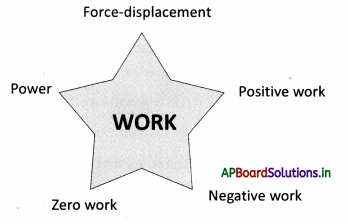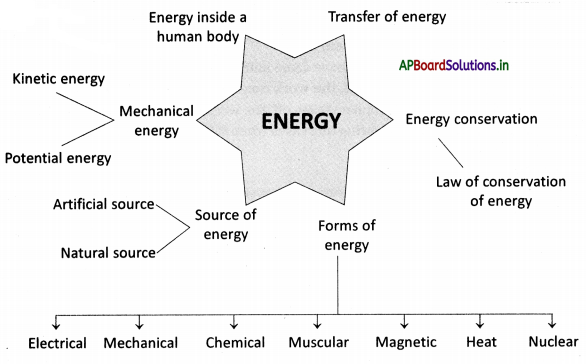# AP Board 9th Class Physical Science Notes Chapter 10 Work and Energy

Students can go through AP State Board 9th Class Physical Science Notes Chapter 10 Work and Energy to understand and remember the concept easily.

## AP State Board Syllabus 9th Class Physical Science Notes Chapter 10 Work and Energy

→ Two conditions need to be satisfied in order to say that work has taken place. One is a force that should act on the object and another is the object must be displaced or there must be a change in the position of the object.

→ Work W = F × s (This formula for work is used in only translatory motion of the object)

→ Units of work ‘N-m’ (or) ‘Joule’.

→ Work has only magnitude, no direction. So work is a scalar.

→ If a force acting on an object and displacement are in opposite directions, then the work done by the force is taken as negative.

→ If work has a positive value, the body on which the work has been done would gain energy.

→ If work has a negative value, the body on which the work has been done losses energy.

→ The capability of doing work by an object is defined as the energy of the object.

→ The energy of an object depends on the position and state of the object which is doing work.

→ Whenever work has been done on an object, its energy either increases or decreases,
i. e., energy transfer takes place from one object to another object.

→ The sun is the biggest natural and primary source of energy.→ Energy can exist in several forms like mechanical energy, light energy, thermal energy, sound energy, electrical energy, magnetic energy, etc.

→ The main source of energy for the human body is food.

→ The energy possessed by an object due to its motion is called kinetic energy.
K.E = $$\frac{1}{2}$$mv2

→ The K.E. of an object increases with its speed.

→ The energy possessed by an object because of its position or shape is called its potential energy.
P.E. = mgh

→ The sum of the kinetic energy and the potential energy of an object is called it’s mechanical energy.

→ Energy will be converted from one form to other forms in nature.

→ The law of conservation of energy states that the energy neither is created nor destroyed. It can only be changed from one form to another.

→ Power is defined as the rate of doing work or the rate of transfer of energy.
Power (P) = $$\frac{\text { Work }(W)}{\text { Time }(t)}$$

→ The unit of power is ‘watt’.→ Work: A work is said to be done when the following conditions are satisfied.

• A force should act on the object
• The object must be displaced or there must be a change in the position of the object

(or)
Work is equal to the product of the force applied and displacement. W = F × s

→ Energy: The capability of doing work by an object is known as the energy of the object.

→ Transfer of energy: Energy transfer will take place from one object to another object when work has been done by the object.

→ Sources of energy: Sun is the main natural source of energy. There are so many derived sources of energy due to the sun. Some artificial sources of energy are thermal energy, electrical energy, etc.

→ Conservation of energy: Energy can neither be created nor destroyed. It can only be changed from one form to another. This is called the law of conservation of energy.

→ Kinetic energy: The energy possessed by an object due to its motion is called kinetic energy.
K.E. = $$\frac{1}{2}$$mv2

→ Potential energy: The energy possessed by an object because of its position or shape is called its potential energy.
P.E. = mgh

→ Mechanical energy: The sum of kinetic energy and the potential energy of an object is called it’s mechanical energy.→ Gravitational potential: An object increases its energy when it is raised through a height, because of the work done on the object against gravity acting on it. The energy of such an object is known as gravitational potential energy.

→ Power: Power is defined as the rate of doing work or the rate of transfer of energy.→ Gaspard-Gustave Coriolis:

• Coriolis was born in Paris on 21 May, 1792.
• He was a French mathematician and physicist.
• He worked to extend the notion of kinetic energy and work to rotating systems.